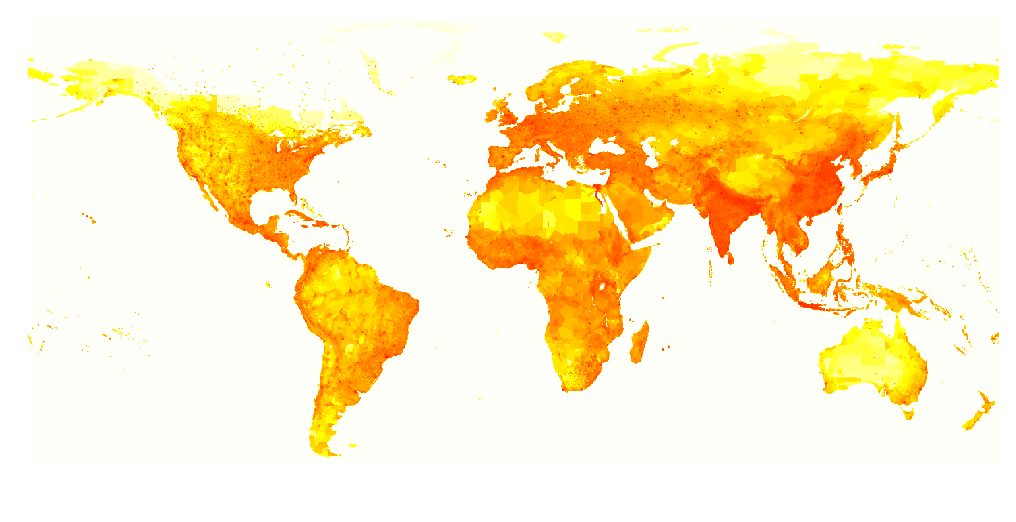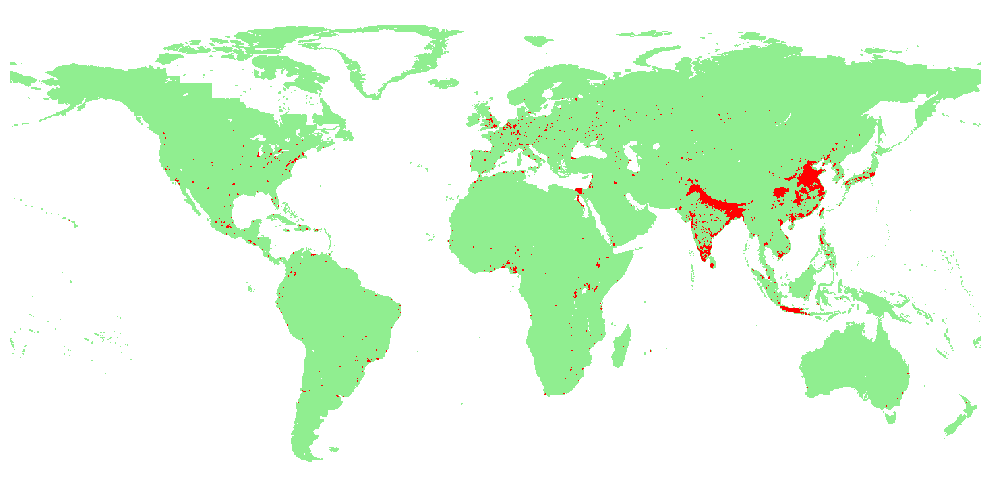Want to share your content on R-bloggers? click here if you have a blog, or here if you don't.

Following my previous post, I wanted to use another dataset to visualize where people live, on Earth. The dataset is coming from sedac.ciesin.columbia.edu. We you register, you can download the database

`> base=read.table("glp00ag15.asc",skip=6)`

The database is a ‘big’ 1440×572 matrix, in each cell (latitude and longitude) we have the population

```>  X=t(as.matrix(base,ncol=1440))
>  dim(X)
 1440  572```

The dataset looks like

```> image(seq(-180,180,length=nrow(X)),
+ seq(-90,90,length=ncol(X)),
+ log(X+1)[,ncol(X):1],col=rev(heat.colors(101)),
+ axes=FALSE,xlab="",ylab="")```Now, if we keep only place where people actually live (i.e. removing cold desert and oceans) we get

```> M=X>0
> image(seq(-180,180,length=nrow(X)),
+ seq(-90,90,length=ncol(X)),
+ M[,ncol(X):1],col=c("white","light green"),
+ axes=FALSE,xlab="",ylab="")```Then, we can visualize where 50% of the population lives,

```> Order=matrix(rank(X,ties.method="average"),
+ nrow(X),ncol(X))
> idx=cumsum(sort(as.numeric(X),
+ decreasing=TRUE))/sum(X)
> M=(X>0)+(Order>length(X)-min(which(idx>.5)))
> image(seq(-180,180,length=nrow(X)), + seq(-90,90,length=ncol(X)), + M[,ncol(X):1],col=c("white",
+ "light green",col="red"), + axes=FALSE,xlab="",ylab="")```50% of the population lives in the red area, and 50% in the green area. More precisely, 50% of the population lives on 0.75% of the Earth,

```> table(M)/length(X)*100
M
0          1          2
69.6233974 29.6267968  0.7498057```

And 90% of the population lives in the red area below (5% of the surface of the Earth)

```> M=(X>0)+(Order>length(X)-min(which(idx>.9)))
> table(M)/length(X)*100
M
0         1         2
69.623397 25.512335  4.864268
> image(seq(-180,180,length=nrow(X)),
+ seq(-90,90,length=ncol(X)),
+ M[,ncol(X):1],col=c("white",
+ "light green",col="red"),
+ axes=FALSE,xlab="",ylab="")```